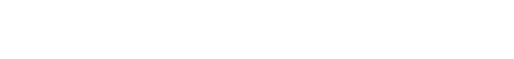## Python Keras MNIST 手写辨识

```from keras.datasets import mnist

(train_images, train_labels), (test_images, test_labels) = mnist.load_data()```

images 是用来训练与测试的资料，label 则为每一笔影像资料对应的正确答案，每一张手写图片都是 28 x 28 的灰阶 Bit Map，透过以下 Python 来看一下资料集的结构

```train_images.shape
len(train_labels)
train_labels
test_images.shape
len(test_labels)
test_labels```## 建立準备训练的神经网路

```from keras import models
from keras import layers

network = models.Sequential()

```network.compile(optimizer='rmsprop',
loss='categorical_crossentropy',
metrics=['accuracy'])``````fix_train_images = train_images.reshape((60000, 28 * 28)).astype('float32') / 255
fix_test_images = test_images.reshape((10000, 28 * 28)).astype('float32') / 255```

```from keras.utils import to_categorical

fix_train_labels = to_categorical(train_labels)
fix_test_labels = to_categorical(test_labels)```

## 开始训练 MNIST 类神经网路

```result = network.fit(
fix_train_images,
fix_train_labels,
epochs=20,
batch_size=128,
validation_data=(fix_test_images, fix_test_labels))``````test_loss, test_acc = network.evaluate(fix_test_images, fix_test_labels)
print('test_loss:', test_loss)
print('test_acc:', test_acc)```## 透过 Keras 图表协助分析训练过程

```history_dict = result.history

loss_values = history_dict['loss']
val_loss_values = history_dict['val_loss']
epochs = range(1, len(loss_values) + 1)

import matplotlib.pyplot as plt
plt.plot(epochs, loss_values, 'bo', label='Training loss')
plt.plot(epochs, val_loss_values, 'b', label='Validation loss')
plt.title('Training and validation loss')
plt.xlabel('Epochs')
plt.ylabel('Loss')
plt.legend()

plt.show()``````plt.clf()
acc = history_dict['accuracy']
val_acc = history_dict['val_accuracy']

plt.plot(epochs, acc, 'bo', label='Training acc')
plt.plot(epochs, val_acc, 'b', label='Validation acc')
plt.title('Training and validation accuracy')
plt.xlabel('Epochs')
plt.ylabel('Accuracy')
plt.legend()

plt.show()```